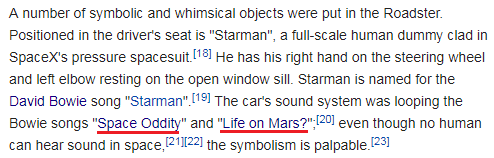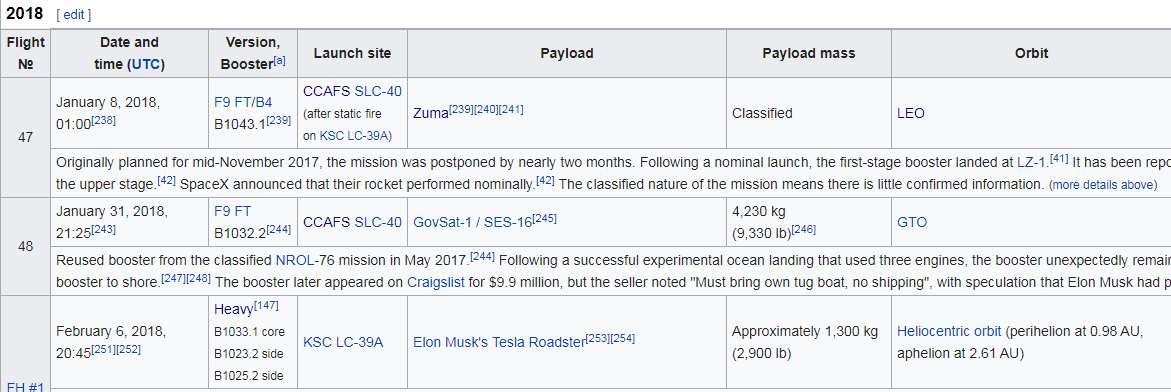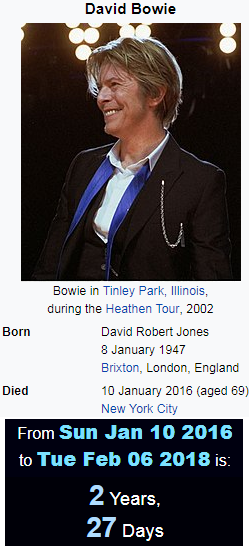## Cipher## Feb 7, 2018

### SpaceX launches Tesla roadster into space

On February 6th we saw "Falcon heavy" launch with "Tesla roadster" and a dummy driver behind the wheel called "Starman"
They chose the perfect song for this trip

Starman = 8+7+8+9+5+8+4 = 49 (Reverse Reduction)CNN fails to mention that song "Life on Mars" is being played as well
Life on Mars = 3+9+6+5 + 6+5 + 4+1+9+1 = 49 (Full Reduction)
Mars is often referred to as the "Red Planet" = 49 (Reverse Full Reduction)

Also this was the 49th SpaceX rocket launchMeasuring from David Bowie's death to this rocket launch is 2 years and 27 days (49th prime number is 227)

SpaceX = 8+11+26+24+22+3 = 94 (Reverse Ordinal)
David Bowie = 4+1+22+9+4 + 2+15+23+9+5 = 94 (English Ordinal)

This level of mocking is unbelievable